### Correction: Does 1+2+3+4+ . . . =-1/12? Absolutely Not! (I think)

(If you'd rather just know what 1+2+3+4+ . . .actually is equal to, check out our next post in this series)

Brief Summary: 1+2+3+4+ . . . is not equal to -1/12, but both the infinite series and the negative number are associated with each other in a way that can be seen in this graph

The area of the little region below the horizontal axis equals -1/12, and the infinite area under the curve on the right gives you 1+2+3+4+. . . , which goes to infinity as you add terms, not to -1/12.

(Update 2-6-14: of course, this graph shows what it looks like if you can only see a finite region of the graph. So 1+2+3+4+...+m is going to infinity, provided m is some finite number. This doesn't say anything about what happens if you could see all the way to infinity - I'd need a much bigger screen for that. For this reason, I am crossing out all the things that I am not so sure about in this post.)

That's a relief - the world makes sense again.

For a longer explanation, read on . . .

(First of all, I want to say I'm sorry to all the mathematicians whose heads exploded when I used non-mathematical reasons to justify why I believed 1+2+3+4+ . . . =-1/12. I was wrong, and after doing some actual math and pondering pretty pictures, I see why.)

Thanks in large part to the patience and persistence of people like Bernd Jantzen, who commented extensively on my previous post on this subject, I have found a way to simply, and graphically explain how the series 1+2+3+4+ . . .  is associated with (and not equal to) the number -1/12, (update 2-6-14: provided you are actually taking the limit as you add terms and not looking at all the infinite terms at the same time as the Numberphiles did).

I usually try to avoid writing equations on this blog, so you're going to have to bear with me. But at least there will be pictures, and those are the most important parts.

Here we go . . .

I find it easier to understand things visually, so it occurred to me to plot out the series 1+2+3+4+ . . . at various points as you add the numbers. These points are called partial sums. This is what you get if you stop the sum after each step

for n=1 the partial sum is 1
for n=2 the partial sum is 1+2 = 3
for n=3 the partial sum is 1+2+3=6
for n=4 the partial sum is 1+2+3+4=10
for n=5 the partial sum is 1+2+3+4+5=15
etc.

If you draw the first five terms on a piece of paper, it looks like this

But you don't have to add all those numbers to calculate value at each point. The numbers, it so happens, are a sequence (1,3,6,10,15, . . .) that you can calculate with a simple formula called a generating function. In this case the generating function is

G(n)=n(n+1)/2

To get a number at any point in the sequence, like say the 5th spot, just plug in 5 for n, and you get the answer

for n=5, G(5)=5(5+1)/2=15

As it turns out, you can also use the generating function to figure out what the values would be between whole numbers. Essentially, you replace the number n with an x, which can have any numerical value you like. If you plot the result, you get this, for positive x.

The curve you see here goes up to infinity as x goes to infinity. So far, so good. But if we're going to plot the graph between the various values of n, we might as well look at negative values of x too.

When you do that, you get a graph like this

There are three interesting areas in this graph. The area above the horizontal axis and to the right of the curve (let's call it A), the area above the horizontal axis and to the left of the curve (call it B), and the area trapped between the curve and the horizontal axis (which I call C).

A and B are large areas - infinite actually, as you extend the curves to infinity, but C is small. In fact, if you use calculus to determine it's size, it's 1/12.

And because it's below the axis, it's conventionally considered negative, so it's -1/12.  It's an easy integral to do, but in case you're feeling lazy, I did it on Wolfram Alpha for you, just click here.

Interesting, isn't it? The curve generated using the partial values of the series 1+2+3+4+ . . . gives you a graph with a little region in it that has an area of -1/12. Hmmmmm.

This is how 1+2+3+4+ . . . and -1/12 are associated. They aren't equal (update 2-6-14: provided you are taking limits), but -1/12, in the form of area C, is a characteristic of the curve While 1+2+3+4+ . . is the series that generates the curve in the first place.

So, despite my previous, non-mathematical argument to the contrary . . .

Update 2-6-14: The limit as m goes to infinity of 1+2+3+4+ . . .+m does not equal -1/12.

As I see it, -1/12 is a kind of label for the curve that you can generate using partial sums of 1+2+3+4+ . . .

The same thing works for 1+2^3+3^3+4^3+ . . . , and  1+2^5+3^5+4^5+ . . .  and so on for any odd power (i.e., zeta(-3), zeta (-5), etc). I used this method to calculate the associated values of the zeta function for powers up to 13. In each case, you get a specific value the area C that's associated with the zeta function that creates the curve.

Here's a list of the C areas I calculated for curves generated by several series

zeta(-1) = 1+2+3+4+ . . . ---> -1/12
zeta(-3) =1+2^3+3^3+4^3+ . . . ---> 1/120
zeta(-5) =1+2^5+^5+4^5+ . . . ---> -1/252
zeta(-7) =1+2^7+3^7+4^7+ . . . ---> 1/240
zeta(-9) =1+2^9+3^9+4^9+ . . . ---> -1/132
zeta(-11) =1+2^11+3^11+4^11+ . . . ---> 691/32760
zeta(-13) =1+2^13+3^13+4^13+ . . . ---> -1/12

They agree with the published values of the zeta function for negative integers listed on Wikipedia.

(Although Wikipedia stops at zeta(-7) and I go to zeta(-13). The fact that the number associated with zeta(-1) and zeta(-13) are the same looks like potential trouble, BTW - after all, how would you know if your -1/12 is associated with zeta(-1) or zeta(-13)? It also suggests that the Numberphiles could have shown that -1/12 = 1+2^13+3^13+4^13+ . . ., or that 1+2^13+3^13+4^13+ . . .= 1+2+3+4+ . . ., if they felt like it.)

This little procedure works for even powers too, except the answer is always zero. Here's what the C area looks for for the curve generated from zeta(-2)=1+2^2+3^2+4^2+ . . .

The parts above and below the axis cancel for all series of this type with even powers, and as a result the total area doesn't give you any information. As you can see for the integral of the curve that comes from1+2^2+3^2+4^2+ . . .

How Could I Have Screwed Up So Badly?

So, I was wrong. 1+2+3+4+ . . . and -1/12 have a relationship with each other but certainly don't equal each other (Update 2-6-14: that is, the series doesn't converge to -1/12, but I am still intrigued by the possibility, suggested by the Numberphiles, that it truly equals -1/12 if ALL the terms out to infinity are included in the sum. Although I confess that I can't really understand what that means).

I'm going to blame my misadventure on the trouble with using words to describe mathematical ideas. Before working out this problem, there was no way I could understand what it means when someone says that the value of -1/12 "can be assigned to an infinite series." They sounded like gibberish, but now I think I get it.

Ramanujan is to blame a bit too. After all, how are we supposed to understand what he was trying to say here?

"I told him that the sum of an infinite number of terms of the series: 1 + 2 + 3 + 4 + · · · = −1/12 under my theory. If I tell you this you will at once point out to me the lunatic asylum as my goal."
-S. Ramanujan in a letter to G.H. Hardy

Are we supposed to realize that "under my theory" means that "=" doesn't mean equal?

I haven't found his original work, but several people have reproduced a calculation by Euler that uses an equal sign in the same way. If the two sides aren't equal then, as I recall from second grade math, you can't use an equal sign.

At least one person I spoke to said that in order to understand it, you have to know what the ". . ." in the expression 1+2+3+4+. . . means. As you can see from the example above, the dots mean exactly what they always mean. If they didn't, then we'd be in almost as much trouble as having equal signs that don't mean equal.

Finally, there are the physicists that say they need the relation 1+2+3+4+ . . . = -1/12. Some of them seem to believe the equation is what it is, and that our failure to understand it shouldn't stand in the way of using it. That struck me as the most romantic view, and it was the one I latched onto at the end of the day. Now, I realize that this is a far too mysterious view.  It's interesting, but I hope this post shows that it's really not that hard to understand the connection between 1+2+3+4+ . . . and -1/12.

While 1+2+3+4+ . . . = -1/12 is clearly not true at all (update 2-6-14: if you insist that the statement is taking a limit) I can see that using the relationship between them might give you a handy way to do things like subtract infinities in consistent ways. The number -1/12 is sort of like a leash that's attached to the infinities in the curve at the top of this post. As long as you hold on to the leash, and don't lose track of the infinities you're dealing with, then you can use them to tame some important physics problems - like those in QED and string theory. How that's done, exactly, is beyond me at this point. But I can sort of imagine what's going on.

I hope there are other, comparably bizarre mathematical controversies out there. One thing is for sure, though, I'm not going to rely on words, even from the most decorated experts in their fields, to try to understand things like this. I'm going to get out a pencil, fire up Wolfram Alpha, and just do the math.

1.there is absolutely no substitute for the henscratches. people who say or do otherwise are cranks.

1.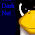2.Ronnie JohanssonMay 3, 2016 at 6:05 AM

Please delete "Dr Ebute"'s fraudish nonsense.

2.Hey Buzz,

Pretty sweet.

1.Thanks, I hope it atones for my earlier transgressions.

2.Hey Buzz, I truly am a Math illiterate but have been fascinated by the seeming tension between the Math and Physics communities over the liberties taken by exploratory Physics that the video revealed. All very entertaining. But this hardly qualifies me to pass any judgement over any transgressions.

I had been pushing my way through some rather dense (for me) Carl Bender lectures just to try, through some sort of magical osmosis, get a better handle on the issue. a futile exercise in many ways. It was really refreshing to come here and have you map it out in such a visual manner, one that a pleb like me could fathom.

So I sauntered off to the couple of discussions I was engaged in to say I was now prepared to concede fully that 1+2+3+4+5... did not equal -1/12th.

But last night, under the influence of a cheap but decent Spanish Red I thought a little more about what you had posted and now I am not so sure, so could I ask you to tell me why you haven't proved the darn sucker.

I mean if you subtract the area A from area B you are left with area C. Does it matter when A and B start on the axis as long as one area is entirely positive and one is entirely negative? Are they not comparatively exactly the same size? And then doesn't that leave you with your -1/12th.

So if the sum was modified and written like this (not even sure if it is allowed but here goes) ...1+2+3+4+5...=-1/12 are we then okay so to speak?

My apologies if this is an stupid question but if you could put a hungover Aussie's mind to rest it would be appreciated.

3.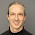The crucial point in the comparison between the areas A and B is the following:

If the two integrals yielding the areas A and B were both convergent, i.e. if the areas A and B had finite values, then it would not matter at all whether the curve is centered around -1/2 (as it is) or around 0. Then one could actually shift the curve a little to the right, centering it around 0, without changing the areas A and B, as infinity is not changed by adding a finite number to it. And then it would be clear that A and B would have to be exactly equal to each other: A - B = 0.

But the two integrals yielding the areas A and B are divergent, so we cannot simply shift the curve and claim that A = B. Instead, neither A, nor B, nor even A-B are finite, they all correspond to divergent integrals. The parametrization of such divergent integrals is crucial when comparing them. It is not possible to simply compare A and B, instead you must specify that the areas you want to compare extend to the same finite number (up to the sign) on the left- and right-hand side, with this number going to infinity in your limit.

Such difficulties with divergent series or integrals make it complicated to relate our naive, non-expert's notion of areas etc. to what the true mathematical outcome is.

4.Hey Bernd,

Thanks a lot for taking the time to reply. I happy to take that all on board. I'm just wondering where does that leave the following statement from Buzz?

“The area of the little region below the horizontal axis equals -1/12, and subtracting the infinite area under the curve on the left from the one on the right gives you 1+2+3+4+. . . , which goes to infinity as you add terms, not to -1/12.”

Are you saying this is not a valid thing to do?

5.Hello Cre Master,

I now realize that you wrote in your previous comment above:
"I mean if you subtract the area A from area B you are left with area C."
That is wrong. As Buzz explained, the difference between the areas A and B increases beyond limits (approximately as fast as the infinite sum 1+2+3+4+...). In contrast, the area C is finite: -1/12.

Instead, what you cite from Buzz, is (approximately) correct. Buzz always compares finite numbers: He defines the area A as extending from 1 out to some large number n, and the area B as extending from -1 out to the (negative) number -n. It is important that the same limiting number n is used for both areas A and B, otherwise the result would be different. In the end, Buzz looks what happens for larger and larger n and concludes that the difference between the areas A and B grows as fast as 1+2+3+...+n in the limit of large n (or n going to infinity).

Following Buzz' definition, the area A (between 1 and n) is given by A(n) = (2n^3+3n^2-5)/12. And the area B (between -n and -1) is given by B(n) = (2n^3-3n^2+1)/12. So Buzz' difference of areas is A(n)-B(n) = (n^2-1)/2. Compare this to the partial sum G(n) = 1+2+3+...+n = (n^2+n)/2. For large n, the leading quadratic term is equal, but still their difference G(n) - (A(n)-B(n)) = (n+1)/2 grows linearly with n; in particular it does not get small. So the difference between the two areas is not exactly 1+2+3+...+n, but only to leading (quadratic) power in n. Buzz, however, in his comparison (with Wolfram Alpha) calculated the ratio of the two:
(A(n)-B(n)) / G(n) = (n^2-1) / (n^2+n) = (1-1/n^2) / (1+1/n),
and this tends to 1 as n goes to infinity. That is how Buzz concluded that the difference between the two areas grows as fast as 1+2+3+...+n (although this is only approximately true).

If Buzz had defined the two areas differently, say area A from 0 to n and area B from -(n+1) to -1, i.e. both areas starting at the point where G(x)=0 and extending to the right and left for an equal length n, then he would have obtained A(n) = B(n) = (2n^3+3n^2)/12, so two areas of equal size.

Personally, instead of taking the difference between the areas A and B, I would rather have emphasized the relation between the curve G(x) itself (which exactly *equals* the partial sum 1+2+3+...+n at x=n) and the area C (i.e. the finite value -1/12 which one may assign to the infinite sum).

3.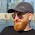"I'm going to get out a pencil, fire up Wolfram Alpha, and just do the math." If you're happy to trust Wolfram products then type this into Mathematica:

Sum[n, {n, 1, Infinity}, Regularization -> "Dirichlet"]

1.Well, I tried it out: Typing
Sum[n, {n, 1, Infinity}, Regularization -> "Dirichlet"]
in Mathematica yields:
-1/12

But that is no surprise! If you look at the definition of what Mathematica does when you tell it to use "Dirichlet" regularization (http://reference.wolfram.com/mathematica/ref/Regularization.html), you get explained that it calculates the regularized sum
Sum[n / n^s, {n, 1, Infinity}],
which includes the regularization parameter s and yields zeta(s-1),
and then takes the limit s->0 of its result analytic function, i.e. of the resulting zeta function, where Mathematica of course is aware of the analytic continuation.

This is exactly the relation I have tried to emphasize in my comments to the previous blog post by Buzz: As soon as you specify what your regularization procedure is, you may get a finite result from such a divergent series. But this result is then not equal to the original sum, it just follows from it when using a certain regularization.

4.Hello Buzz, it's nice that we finally agree in how the relationship between 1+2+3+4+... and -1/12 can be seen. I must confess I almost gave up trying to convince you, and if this wasn't a blog hosted on an APS website (I know APS as author and reviewer in its journal Physics Review D), I would probably not have wasted that much effort.

For me, the way you now have come up for relating the divergent series and the finite value is not the easiest way to see it. But if it helps others, who are not familiar to concepts like analytic continuation, that's fine.

5.Buzz lists above the values of the zeta function for several negative integer arguments, stating that these correspond to the "C areas" of the functions arising from the partial sums of the corresponding series. Why is this so?

Consider the infinite sum 1^p + 2^p + 3^p + ... with its partial sum

S_p(n) = 1^p + 2^p + 3^p + ... + n^p.

Buzz provided S_p(n) in the case p=1: S_1(n) = n(n+1)/2. The general result is indicated e.g. on the Wikipedia page about Bernoulli polynomials (http://en.wikipedia.org/wiki/Bernoulli_polynomials#Sums_of_pth_powers):

S_p(n) = ( B_{p+1}(n+1) - B_{p+1}(0) ) / (p+1),

where B_{p+1}(x) is the (p+1)th Bernoulli polynomial.
This relation is correct for integers p>0, and in this case we have S_p(0) = 0 as it should, because B_{p+1}(1) = B_{p+1}(0) = B_{p+1}, the (p+1)th Bernoulli number, for p>0. But also S_p(-1) = 0, because B_{p+1}(0) is subtracted from itself then. So the function S_p(x) (with x instead of n) has zeros at least at x=-1 and at x=0.

Examples:
S_1(x) = x (x+1) / 2,
S_2(x) = x (x+1) (x+1/2) / 3,
S_3(x) = x^2 (x+1)^2 / 4,
S_4(x) = x (x+1) (x+1/2) (x^2+x-1/3) / 5.
Starting from p=4, these polynomials have also zeros outside the interval [-1,0]; e.g. S_4(x)=0 for x=0, -1/2, -1, -1.26..., and +0.26... So the finite areas between the curve S_p(x) and the x-axis are not fully covered by those between -1 and 0.

For obtaining the "C areas", Buzz integrated these functions S_p(x) from -1 to 0. As said above, this does not cover all finite areas under the curve S_p(x), starting from p=4. Still, let us do what Buzz did to obtain the "C areas".
The Bernoulli polynomials B_p(x) (starting from p=1) have the property that their integrals from 0 to 1 yield zero (see http://en.wikipedia.org/wiki/Bernoulli_polynomials#Representation_by_an_integral_operator). For us this means that the integral over B_{p+1}(x+1) from -1 to 0 vanishes. So the "C areas" get contributions only from the second, constant term -B_{p+1}(0)/(p+1), integrated over [-1,0]:

C areas = -B_{p+1}(0) / (p+1) = -B_{p+1} / (p+1),
with the Bernoulli number B_{p+1}.

But these are exactly the values of the zeta function for negative integers, obtained via analytic continuation (see http://en.wikipedia.org/wiki/Riemann_zeta#Specific_values):

C areas = zeta(-p)= -B_{p+1} / (p+1), for integer p>0.

For larger arguments, a representation of the zeta function is given by the infinite sum

zeta(s) = 1^(-s) + 2^(-s) + 3^(-s) + ..., for (real part of) s>1,

which formally (!) reproduces the original series for s=-p.

Summarizing:
The partial sums of the divergent series 1^p + 2^p + 3^p + ... are given by the function S_p(x) with x=n. Through analytic continuation of the zeta function, the divergent series is related to zeta(-p) which is equal to the sum of finite areas under the curve S_p(x) between its zeros at -1 and 0.

This is one way to relate the divergent series to zeta(-p). But, as Buzz emphasizes, keep in mind that the infinite sum 1^p + 2^p + 3^p + ... itself does not have a finite value (for p>=-1) and is not equal to zeta(-p).

6.Hey Buzz, it's a pity that you have now reverted to your previous viewpoint instead of accepting that 1+2+3+4+... simply is not equal to -1/12 (although the two are related to each other).

So you are now claiming: "I still believe 1+2+3+4+ . . .= -1/12, if the ENTIRE infinity of whole numbers are included" (cited from your updates of Feb 6th above).

But what do you actually mean by "1+2+3+4+... with the entire infinity of whole numbers included"? No mathematical definition exists of what your statement should mean.

You obviously don't mean adding consecutively more and more of these numbers, because this standard definition of the value of an infinite sum diverges here and does not yield a finite number.

Do you mean "1+2+3+4+..." understood in the sense of Ramanujan summation or zeta-function regularization? Then the result is indeed -1/12. But neither Ramanujan summation nor zeta-function regularization actually yield the sum of infinitely many terms of a divergent series. They are simply procedures to treat divergent sums and assign them finite values in a consistent and meaningful way. Both of these procedures actually use analytic regularization to drop in a well-defined way the divergent and infinitely positive contribution originating from the upper end of the summation. So that's how both procedures find the negative result -1/12 from what originally was a sum of purely positive numbers. But, when you look at how Ramanujan summation or zeta-function regularization obtain this result, you may convince yourself that this result is not equal to the sum of the infinitely many numbers of the original series, but that it follows from this series in a more complex and intricate way.

Simply stating "1+2+3+4+... with the entire infinity of whole numbers included" does not mean anything in mathematics. It only means something if you already have a certain regularization procedure in the back of your mind.

Anyway, I have the impression that further debates here are useless. You probably cannot understand what I have tried to explain through my many comments. So I will stop wasting my time any longer.

7.Hi Buzz,

It takes a brave man to admit when he has got something wrong but to be now admitting your 'wrongness' is not as wrong as first thought must have been a mission, so well done.

However could I bother you for a small clarification? When you said "And of course, the difference between area A an B is itself infinity if you extend the curves out infinitely." I assume that you are not taking about the area of region A compared to the area of region B since aren't these exactly the same?

8.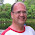For me, not a mathematician, this is a perfect example for that you can prove anything with infinity mathematics.

In my view, infinity does not exist (in the real world), nor does 0.

Infinity = anything / 0

so

0 = anything / infinity.

Physical argument: the smallest thing (measure) is the planck dimension (planck lenght, planck time etc.) so there cannot be an infinite number of since the beginning of time (the big bang).

1.I possess no sheep, therefore I possess 0 sheep. 0 is very much in the real world.

2.No, zero is not in the real world.
Imagine that you have two bags, in one there is one apple and in the other you have one pear. Then we remove the apple and the pear out of the bags, now in one bag you have zero apples and in the other zero pears, but zero apples and zero pears have the very same properties, therefore they must be the same thing, as we know, pears and apples are not equal to each other, so it must be that zero is nothing but a concept that does not exist in the real world.

3.I understand how puzzling it can be. Is zero a quantity or not? Is a negative number a quantity or not? Talking about apples and pears is a little worn out, I think.

So, here:
There are two different bags; let's call them bagA and bagB. They are freshly made, and nothing has ever been put in them before.
Each bag has nothing in it. The bags are in an enclosed vacuum, so there is no air in them and no air outside of them.

There are two different things; let's call them thingZ and thingY.
You put thingZ into bagA, and thingY into bagB.

You then have a bag - bagA - containing thingZ and a bag - bagB - containing thingY.

If you remove thingZ from bagA and thingY from bagB, the bags will then be empty because the things that were in them have been removed.

Because there used to be a thingZ in bagA, and one was taken out, there is a shortage of one thingZ.
BagA therefore contains -1 thingZ.
Likewise, bagB therefore contains -1 thingY.

But all the time there was a thingZ in bagA, bagA also had no thingY.
Similarly, while there was a thingY in bagB, bagB also had no thingZ.
In fact, ever since the bags were made, there was also none of thingX, none of thingW, no tomatoes, onions, apples, bicycles, orang-utans or saxophones in them. None of any other thing, in fact, in either bag. And the same goes for what is outside of each bag either, because it was a vacuum.

If it is true that zero is something that does exist, then zero is a complete lack of stuff. But we know that it wasn't always like that, because there used to be a thingZ and thingY in the bags.

Regarding anything and everything else, there always was a complete lack of anything else in the bags, and everything outside the bags.
Because you cannot remove something that isn't there, bagA still contains zero thingY and bagB still contains zero thingZ - and zero everything else except as was stated in the paragraph before this one. And outside of the bags is still zero everything.

But the bags don't contain zero of everything, they contain -1 of one thing.

Zero is more than -1. The bags therefore collapse under the pressure of absolutely nothing.

4.Interesting, except removing thingZ from bagA doesn't leave you with -thingZ in bagA. to do that you would have to remove 2*thingZ. Otherwise, taking thingZ out of bagA, and then replacing it would leave you with nothing in bagA. But clearly removing thingZ from bagA and replacing it shouldn't make thingZ dissappear.

5.Let's assume I gave you zero fucks. How many fucks have I given you?

9.i think 1+2+3+4+.........=infinity

10.1+2+3+4+5.................= infinity!!!

11.1+2=3+3=6+4=10+5=15+6=21+7=28+8=36+9=45+10=55.......................................=infinity

1.Of course, the partial sums of 1+2+3+4+... are tending to infinity. Nobody claimed anything different. The whole discussion here is about how to assign a meaningful finite value even to a divergent sum like this one. And the discussion is about the question whether one may call this finite number the value of the divergent series or just a meaningful number that one may use in replacement of the series under some conditions.

2.Une somme partielle s'arrête à n : Sn = 1 + 2 +... + n. On ne peut pas appliquer les propriétés de l'addition dans l'expression 1 + 2 + 3.... (sans fin) et c'est la raison pour laquelle on en donne une définition toute faite.
et tant qu'à faire, on choisit évidemment la plus intuitive, mais intuitive ou pas, c'est une définition et la cohérence interne des propriétés des séries numériques tient dans celle des propriétés des limite (quand n -> + infini), un point, c'est tout. Dès lors qu'une autre définition se présente et qu'elle produit un autre espace de raisonnement cohérent, elle est tout aussi valable dans son champ mathématique d'application. Tout prolongement analytique d'une fonction f initialement non définie en un point, qui attribue une valeur en ce point licitement en ce sens que la fonction n'interdit pas de le faire, est fondé. Attribuer la valeur (-1/12) ne pose aucun problème, c'est une définition aussi licite que celle qui consiste à dire que 1 + 2 +.... = lim Sn
(= + infini). Si les gens n'ont pas compris que l'addition est une loir de composition ''interne'' de R dans R ! et non de
R U {infini} dans R U {infini} ! et que par conséquent, toutes les propriétés de l'addition - l'associativité, l'existence d'un élément neutre ''0'', d'un symétrique (-x), la commutativité, la distributivité de la multiplication sur l'addition - n'ont plus de sens lorsque qu'on parle ''d'ajouter'' une infinité de termes, alors, c'est que les gens ne parlent pas de la même opération. Des propriétés dépendent des définitions que l'on donne aux choses, il ne faut croire que la chose a une essence bien définie avant qu'on la définisse nous-même !

12.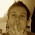This article is superb, I love it. That infinite series thing really perturbed me when I first saw it but I couldn't see how to examine it more effectively such that I could get to the point that I wasn't perturbed by it any more. Watching you do it above now makes me annoyed I didn't have the idea of doing that myself. But the fact is I didn't. So simple, so insightful, so satisfying. Thanks for that and if you could now just solve every other annoying problem for me I would be most grateful ;o)

13.Area A = Area B since they both are infinitely large areas. That series diverges, you learned that in Cal2 or Math Physics of DiffEq. C'mon.

14.Valuable liveliness is also saved which you can set aside to your family or to manually. Completing an Online Distance Learning course gives you more flexibility while studying over conformist classroom set up.

15.Contact a Great man on agbadado@gmail.com or call him on +2347060552255 who help me to solve my problems when my ex boyfriend was blackmailing me after trying many ways to stop him but it didn't work for me until i met this man who help me cast a spell that stop him for blackmailing me and now he is pleading forgiveness and i believe he can solve any problems you are having because he just solve mine.

16.1+2+3+4+... = -1/12 (R) where (R) is the Rumanujan Summation. This is not a normal -1/12. It basically is a categorization of the series in question. It should be read, "the sum of one plus two plus . . . has a Rumanujan summantion of -1/12" (as opposed to "equals -1/12").
http://en.wikipedia.org/wiki/Ramanujan_summation

17.I have posted a message explaining the dilemma.
What has happened here was inevitable, mathematics is not sustainable.

Here is the link to my message: http://marques.co.za/duke/news_win.htm

It will not surprise me if this comment is censored (Moderated)

1.To be more specific, irrational mathematics('mathematics'), is not sustainable.

18.Hi .....
do you wants....idea from amateur...from me or not...

Easy...! Open Mind...

Zeta Functions not importtant ....Harmonic important more!

Zeta function that important Zeta(-1) and Zeta(-3)

I show you....develop zeta in term cartoon.....and blinding set and anti
and i predict somethings in Higher Dimension...You can help me proof

http://www.quora.com/Zeta-1-1-12-really-Sure-1

http://www.quora.com/What-is-the-solution-to-sqrt-1+2-sqrt-2+3-sqrt-3+4-sqrt-4+5-sqrt-5+6-sqrt-6+

sawat

19.The author of this article is absolutely correct to point out that these supposedly mysterious values can be found by calculating the definite integral between 0 and -1 of the expression for the respective sum to the nth term (a.k.a. their partial sum expressions).

This is also mentioned in this interesting 'response' video (it responds to the claims made in the video that is the subject of this discussion): https://www.youtube.com/watch?v=BpfY8m2VLtc

It shows what happens if you do the series manipulations with rigorously (hint - you do not get -1/12) and it explains why other methods get this -1/12 result.

The response video claims this -1/12 result is the result of a mistake. The mistake is one of taking a function that applies to just positive whole numbers, manipulating it in ways that bring decimal numbers and negative numbers into play, and then interpreting the result as though it still relates to positive whole numbers.

1.RZ(-c) is Riemann's Zeta value at -c = I(n=0to -1)[1^c+2^c+3^c+...+n^c]dn
Where I(n=0to-1) is the definite integral from 0 to -1(lower limit)
RZ(-1)=I(n=0to-1)[1+2+3+...+n]dn=I(n=0to-1)[(n/2)(n+1)]dn= -1/12evaluated.
RZ(-13)=I(n=0to-1)[1^13 +2^13 +3^13 +...+n^13]dn
RZ(-13)=I(n=0to-1)[n^14/14+n^13/2+13n^12/12-143n^10+429n^8/84-429n^6/60
+715n^4/132 -691n^2/420]dn= -1/12 on evaluation= -B(14)/14= -(7/6)(1/14)
Note1^c+2^c+3^c+...n^c for odd c values has factors of n(n+1)with zeros at 0,-1.
Anyone interested in an expression for the yet unsolved sum of the positive ODD
Zeta series Z(2n+1) in terms of π^(2n+1)? Alan Walter, Sydney.

2.The term above -143n^10 should be -143n^10/60. Inadvertently the denominator 60 was omitted.Also the term n^c should be +n^c with
a plus sign inserted. Integrating this corrected function from 0 to -1
will now render the result of -1/12 = RZ(-13) = RZ(-1). Alan Walter,Sydney.

20.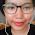How can I find the following cardinalities?
|{{{1},{2{3,4}},Emptyset}}

21.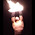Every term of Zeta(-1) is larger than Zeta(1), except the first which are both 1. Since Zeta(1) equals infinity (proven by the comparison test) -> Zeta(-1) is also infinity, due to the comparison test. QED.

22.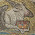In order to use negative numbers for the integral, doesn't that require the problem to be the sum of all natural numbers including the negative ones? The left hand integral area cannot cancel the right hand area otherwise. The sum of all natural numbers from -x to x in the limit as x approaches infinity is -1/12?

23.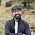What i think actually is the area under the curve calculated by sir cre gives the sum of infinite real numbers between 0 and -1 which has no relation with the sum of infinite NATURAL numbers.

24.25.Saved as a favorite, I love your web site!

26.I was wondering if you ever thought of changing the layout of your website?
Its very well written; I love what youve got to say.

But maybe you could a little more in the way of content so people could connect with it better.

Youve got an awful lot of text for only having 1 or 2 pictures.
Maybe you could space it out better?

27.You are all wrong. The series equals -1/12

Zeta(-1) is the slope (derivative) at the point zero (x=0) of a function f which equals:

f(x) = sum(e^(nx),n,1,infinity)

The future of math and physics is complex 'fractional' calculus and its connection to number theory.

### How 4,000 Physicists Gave a Vegas Casino its Worst Week Ever

What happens when several thousand distinguished physicists, researchers, and students descend on the nation’s gambling capital for a conference? The answer is "a bad week for the casino"—but you'd never guess why.

### Ask a Physicist: Phone Flash Sharpie Shock!

Lexie and Xavier, from Orlando, FL want to know:
"What's going on in this video? Our science teacher claims that the pain comes from a small electrical shock, but we believe that this is due to the absorption of light. Please help us resolve this dispute!"

### The Science of Ice Cream: Part One

Even though it's been a warm couple of months already, it's officially summer. A delicious, science-filled way to beat the heat? Making homemade ice cream.

(We've since updated this article to include the science behind vegan ice cream. To learn more about ice cream science, check out The Science of Ice Cream, Redux)

Over at Physics@Home there's an easy recipe for homemade ice cream. But what kind of milk should you use to make ice cream? And do you really need to chill the ice cream base before making it? Why do ice cream recipes always call for salt on ice?